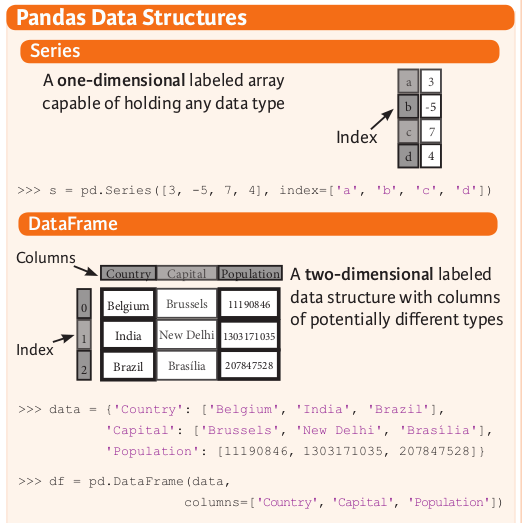2018年12月11日 星期二

拿公投統計資料學 pandas

一、 資料結構與資料概觀Pandas 主要用來處理一維陣列 (在 pandas 文件裡稱為 series) 及二維陣列 (稱為 data frame)。 程式可以用整數註標存取 series 裡的元素 (類似 python 內建的 list)， 也可以用文字標籤存取 (類似內建的 dict)。 不論是整數註標或文字標籤， 通稱為 index。 程式存取 dataframe 裡的元素時， 可以按照 column (欄位) 名稱一次擷取一整直行， 或是按照 index 註標/標籤一次擷取一整橫列。 以上請見 cheatsheet 的 Pandas Data Structures 那一段的兩張圖， 也請先進入 python3 並且照著 Karlijn 的文章建立 `s = pd.Series(...)``df = pd.DataFrame(...)` 兩個變數， 然後就可以測試以下：

• `.shape` 資料有幾列？ 幾行？
• `.index` 資料的所有文字標籤， 或整數註標範圍
• `.columns` (限用於 data frame 類型) 資料的所有欄位名稱
• `.info()` (限用於 data frame 類型) 資料摘要概述
• `.count()` 資料有幾列
• `.describe()` 資料當中每個數字欄位的基本統計資訊： 平均值、 標準差、 最大、 最小、 中位數等等。

二、 準備測試資料

1. 把數字裡的 (每三位一個) 逗點去掉， 同時把引號去掉。
2. 只擷取其中幾個較有趣的欄位。 詳見 perl 的 csv 句型
3. 把 「第xx案」 改成 「cxx」

三、 擷取整欄、 進行四則運算等等

```import pandas as pd
refm['同意票數']
x=refm['同意票數']+refm['不同意票數']-refm['有效票數']
x.describe()
x=refm['有效票數']+refm['無效票數']-refm['投票數']
x.describe()
```

```summary = refm[refm['縣市']=='全國']
summary['同意票數']/summary['投票數']
```

四、 刪除列、 刪除欄

```refm2 = refm[~ refm['鄉鎮市區'].isnull()]
refm3 = refm.dropna(subset=['鄉鎮市區'])
# 上面兩句效果相同。 可以說第二句是第一句的簡寫。
```

```refm = refm3
refm['贊成比例'] = refm['同意票數']/refm['投票數']
refm['鄉鎮市區'] = refm['縣市'] + refm['鄉鎮市區']
refm2 = refm.drop(columns=['縣市', '同意票數', '不同意票數', '有效票數', '無效票數', '投票數', '投票權人數'])
refm3 = refm[['案件', '鄉鎮市區', '贊成比例']]
# 上面兩句效果相同。
```

五、 疊疊樂

```refm = refm3
x07 = refm[refm['案件']=='c07'][['鄉鎮市區','贊成比例']]
x08 = refm[refm['案件']=='c08'][['鄉鎮市區','贊成比例']]
tmp = pd.concat([x07.set_index('鄉鎮市區'), x08.set_index('鄉鎮市區')], axis=1, sort=True)
tmp.columns = ['c07', 'c08']
```

```summary = refm[refm['案件']=='c07'][['鄉鎮市區','贊成比例']].set_index('鄉鎮市區')
cnames = refm['案件'].unique()
for c in cnames[1:] :
tmp = refm[refm['案件']==c][['鄉鎮市區','贊成比例']]
summary = pd.concat([summary, tmp.set_index('鄉鎮市區')], axis=1, sort=True)

summary.columns = cnames
```

六、 用 matplotlib 畫圖

Matplotlib 是傳統的 python 繪圖函式庫。 先前我寫過一個 scatterplot 範例， 包含解決中文問題。 Pandas 的 dataframe 可以直接呼叫 matplotlib 的繪圖函數， 像這樣： `drawing=summary.plot(kind='scatter', x='c07', y='c08')` 但這只是產生一個圖片物件； 要秀在螢幕上， 還必須：

```import matplotlib.pyplot as plt
plt.savefig('ref-07-08.svg')
plt.show()
```

七、 用 plotly 畫圖

```import plotly
import plotly.graph_objs as go
plotly.offline.plot([
go.Scatter(
mode='markers',
x=summary['c07'],
y=summary['c08'],
text=summary.index
)],
filename='ref-07-08.html'
)
```

```import math
N = 60
X=[(float(k)/N-0.5)*math.pi*2 for k in range(N+1)]
Y=[math.sin(x) for x in X]
plotly.offline.plot([go.Scatter(mode='lines+markers', x=X, y=Y)])
```

八、 結語

Pandas 擷取欄位的語法蠻直覺的， 讓寫程式幾乎變得跟說話一樣精簡扼要。 尤其跟 matplotlib 或 plotly 搭配畫圖時， 一句話就把圖畫出來， 很方便。